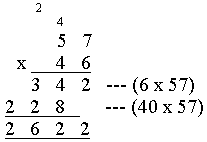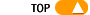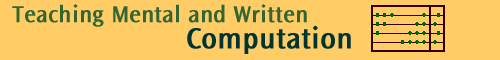Back to Multiply

# Teaching algorithms for multiplication

In the primary school, children are taught multiplication using a formal written method that is based on: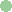the place value systemmultiplication tables up to 10 by 10the distributive property of multiplication over addition.

Understanding the formal written algorithm for multiplication depends on assembling together understanding of several separate steps. Therefore the ideas must be introduced through a number of stages. Students need to be competent and comfortable with each stage prior to moving onto the next stage. Ample experience with place value materials prior to the introduction of symbolic notation will assist children consolidate knowledge at each stage.

The sections below give the reasoning behind the steps of the formal written algorithm, the intermediate forms which teachers use before students learn the most efficient procedure and link to explanations using place value material.

 Learning the standard written algorithm Stage 1: Develop meaning(s) for multiplication (Repeated addition is sufficient for multiplying whole numbers) Stage 2: Multiplication by a single digit Stage 3: Multiplication by ten Stage 4: Multiplication by a multiple of ten Stage 5: Multiplication by numbers with two or more digits Click below to see how to explain multiplication using concrete materials in stages 2 and 3, Multiplication using place value material Other ways of setting out the standard algorithm Other algorithms for whole number multiplication

Stage 2: Multiplication by a single digit

 2 3 x  4

23 is 2 tens and 3 ones.

3 ones multiplied by 4 gives 12 ones and

2 tens multiplied by 4 gives 8 tens (that is 80).

80 and 12 are added to give the final product 92.Children should write multiplication in this form for some time, until the procedure is familiar and the concepts (especially the distributive property) is well understood. Ruling up (or using squared paper) and labeling columns for tens and ones is recommended in the early stages. Later it can be reduced to a more compact form: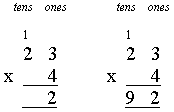The 3 ones are first multiplied by 4 giving the product 12, which is 1 ten and 2 ones. 2 is written in the ones column and the 1 is recorded in the tens column. Now the 2 tens are multiplied by 4 to give 8 tens. The 1 ten recorded before is added on, so the product has 9 tens.

Click here to see how this aspect of multiplication is explained with place value material.

Stage 3: Multiplication by ten

Children must learn how to multiply by multiples of ten. It is very important that they know that to multiply a whole number by ten a zero can be "added to the number", but this is dangerous terminology. Teachers should be aware that many children may fall victim to simply knowing the rule of "adding zeros". It is important that children understand that the effect of the zero is to move digits into the next larger place value column.

10 x 2 = 10 x 2 ones = 2 tens = 20

10 x 152 = 10 x (1 hundred + 5 tens + 2 ones)

= 10 hundreds + 50 tens + 2 tens

= 1 thousand + 5 hundreds + 2 tens

= 1520

Click here to see how this is explained with place value material.

Stage 4. Multiplication by a multiple of ten and power of ten

After learning how to multiply by ten, children can see how to multiply by multiples of ten. This step relies on understanding the associative property of multiplication and understanding. This step is NOT assisted by place value or other concrete materials.

Multiplication by 30 is done by multiplying by 3 and then by 10 (or vice versa). To multiply by 30, first multiply by ten (by putting down the zero) and then by 3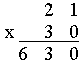To multiply by 300, first multiply by one hundred (by multiplying by ten and then by ten again i.e. putting down two zeros and so moving the digits by two place value columns) and then by 3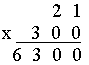Stage 5: Multiplication by a number with two or more digits

These multiplications require understanding of all that has come before. They are less important now that calculators are common so not all children need to practice sufficiently to be able to accurately calculate with very large numbers. Extensive practice is no longer a high priority.

 5 7 x 4 6

To compute this product, 57 is first multiplied by 6 ones and then by 4 tens. The two results are then added to get the final result. It will be written down as follows.Next, 57 is multiplied by 40 (this is done by multiplying by 10, putting down the zero, and then multiplying by 4)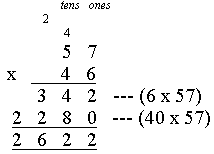Move carry digits somewhere else and move the multiplication sign to the other side.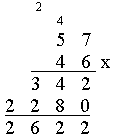Move carry digits somewhere else and place the multiplication sign on top other side.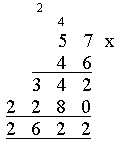Not advised.